# Properties of 3D shapes

## What are 3D shapes?

3D shapes are shapes with three dimensions, such as width, height and depth.

## 3D shape vocabulary

The side of a 3D shape is called the face. 3D shape faces are flat.

The edges of a 3D shape are where the faces meet.

The corners of a 3D shape are called vertices. Vertex is the singular of vertices.

A regular 3D shape’s faces are all equal. A cube is an example of a regular 3D shape. It has six square faces that are all the same size.

An irregular 3D shape has faces of differing shapes and sizes. A cylinder is an example of an irregular 3D shape. It has three faces, two are circles and one is a rectangle.

## What is a polyhedron?

A polyhedron is a 3D shape made up of a collection of flat polygons. Polyhedrons can have any number of faces. Triangular prisms and cubes are examples of polyhedrons.

## 3D shape names and facts

Here is a selection of some of the common 3D shapes and their properties:

## Properties of a sphere

• A sphere is a three-dimensional shape.
• A sphere has 1 curved face.
• A sphere has no vertices.
• A sphere has no edges.
• A sphere is symmetrical.
• A sphere is not a polyhedron.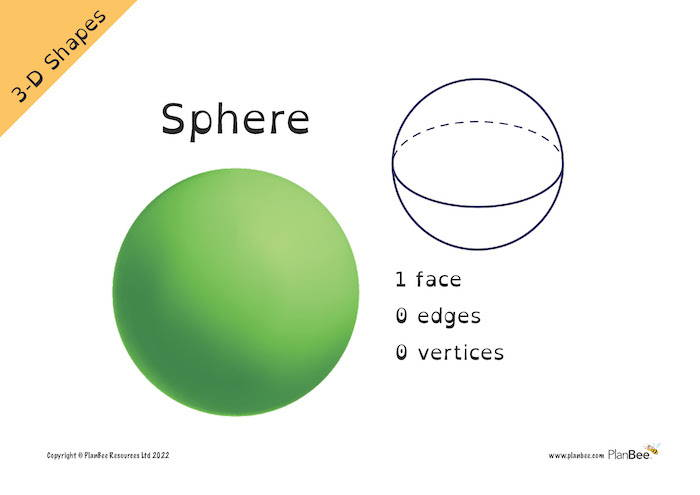## Properties of a hemisphere

• A hemisphere is a three-dimensional shape.
• A hemisphere has 2 faces. 1 curved face and 1 flat circular face.
• A hemisphere has no vertices.
• A hemisphere has 1 edge.
• A hemisphere is half of a sphere.
• A hemisphere is not a polyhedron.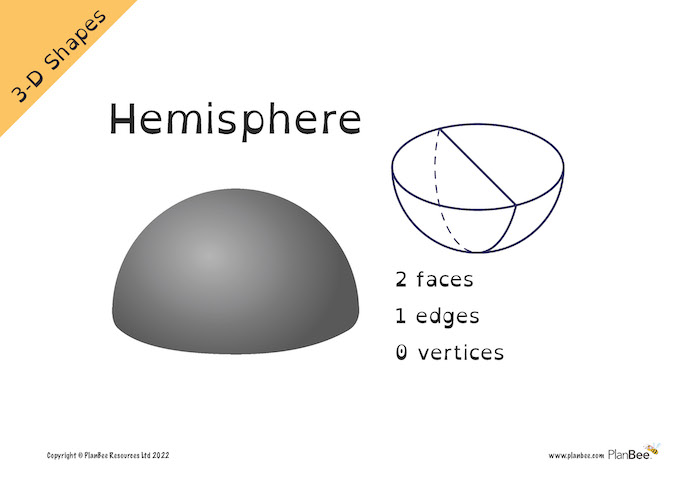## Properties of a cube

• A cube is a three-dimensional shape.
• A cube has 6 flat faces.
• A cube's faces are all squares. They are all the same size and shape.
• A cube has 8 vertices.
• A cube has 12 edges.
• A cube is a polyhedron.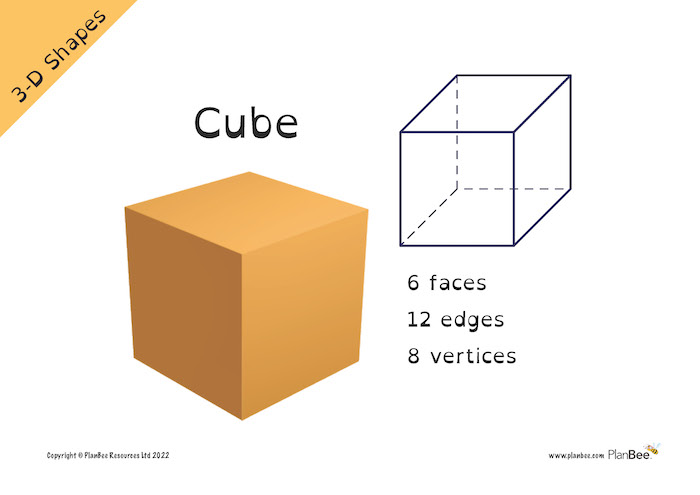## Properties of a cuboid

• A cuboid is a three-dimensional shape.
• A cuboid has 6 flat faces.
• A cuboid's faces are all rectangles.
• A cuboid has 8 vertices.
• A cuboid has 12 edges.
• A cuboid doesn’t have to have regular faces like a cube.
• A cuboid is a polyhedron.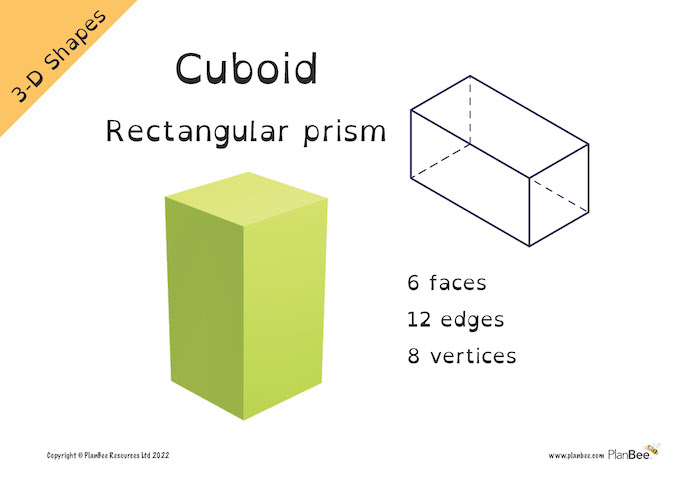## Properties of a cone

• A cone is a three-dimensional shape.
• A cone has 2 faces. One is a flat circular face. The other is a curved face.
• A cone has 1 apex. (The pointed tip of a cone is called the apex.)
• A cone has 1 edge.
• The flat circular face of a cone is the base.
• A cone is not a polyhedron.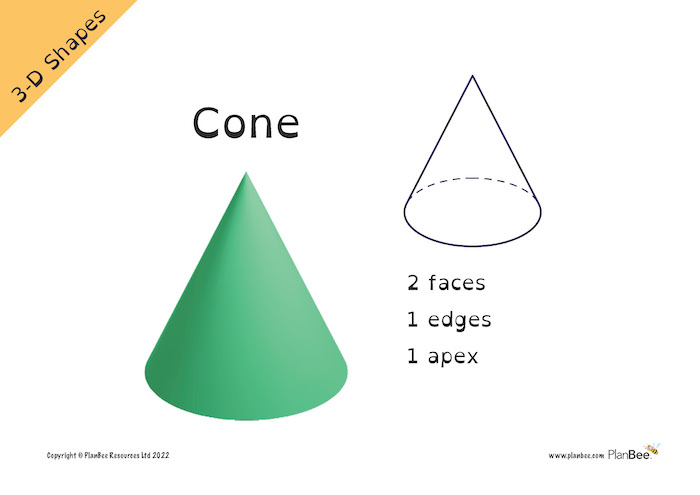## Properties of a cylinder

• A cylinder is a three-dimensional shape.
• A cylinder has 3 faces. Two of the faces are flat circular faces, one is a curved rectangular face.
• A cylinder has 0 vertices.
• A cylinder has 2 edges.
• A cylinder is not a polyhedron.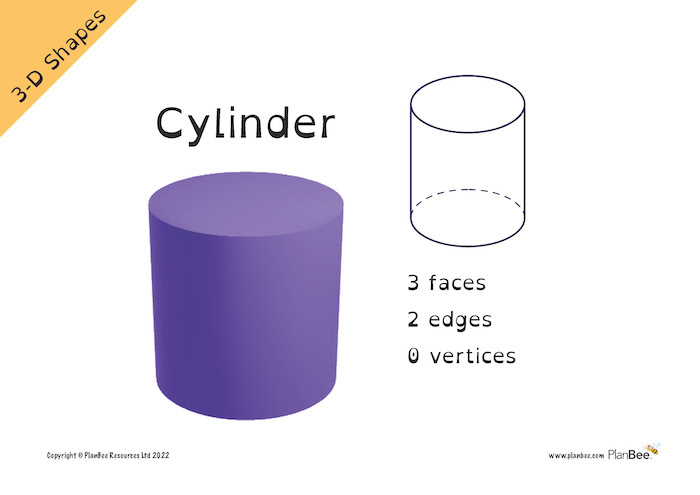## Properties of a triangular prism

• A triangular prism is a three-dimensional shape.
• A triangular prism has 5 faces. Two of the faces are flat triangular faces, three are flat rectangular faces.
• A triangular prism has 6 vertices.
• A triangular prism has 9 edges.
• A triangular prism is a polyhedron.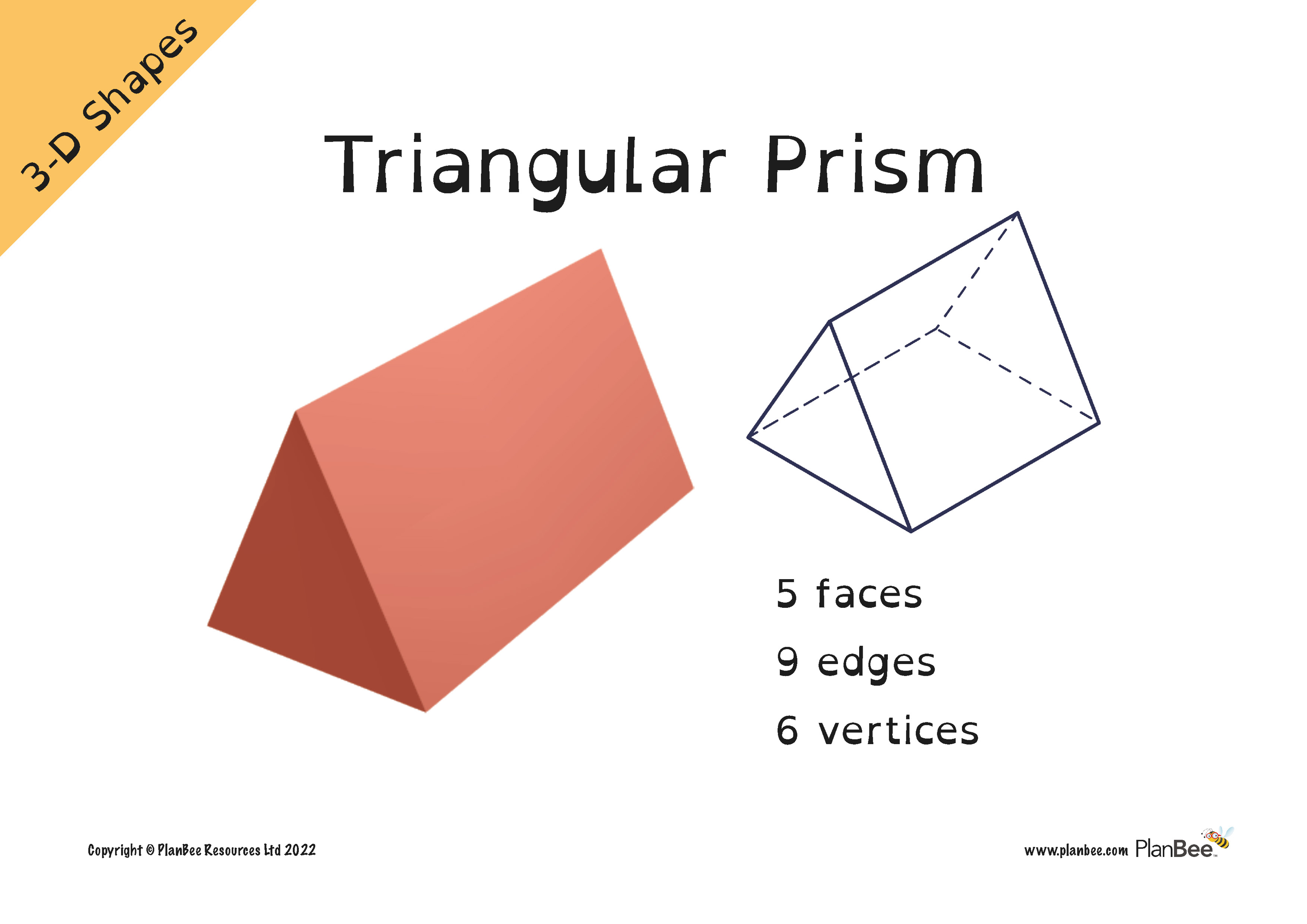## Properties of a pentagonal prism

• A pentagonal prism is a three-dimensional shape.
• A pentagonal prism has 7 faces. Two of the faces are flat pentagonal faces, five are flat rectangular faces.
• A pentagonal prism has 10 vertices.
• A pentagonal prism has 15 edges.
• A pentagonal prism is a polyhedron.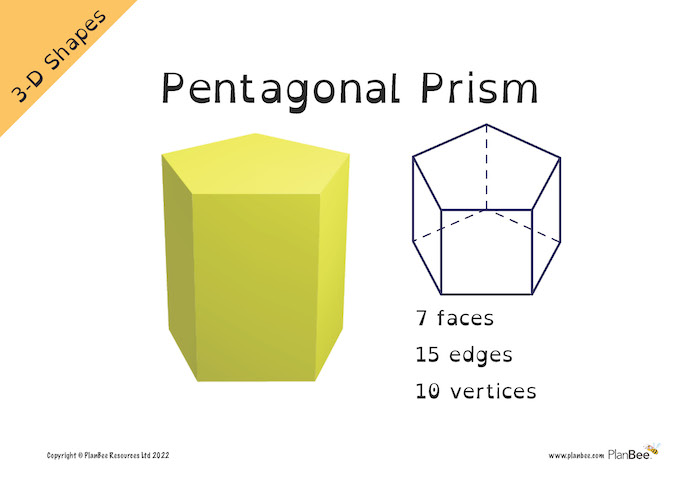## Properties of a hexagonal prism

• A hexagonal prism is a three-dimensional shape.
• A hexagonal prism has 8 faces. Two of the faces are flat hexagonal faces, six are flat rectangular faces.
• A hexagonal prism has 12 vertices.
• A hexagonal prism has 18 edges.
• A hexagonal prism is a polyhedron.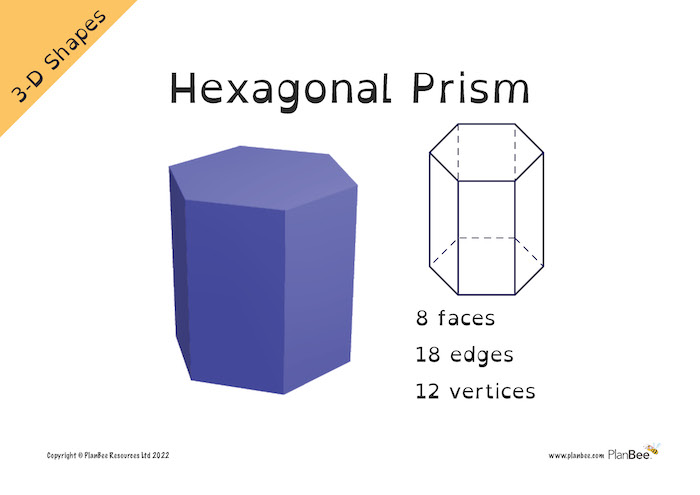## Properties of a triangular based pyramid

• A triangular based pyramid is a three-dimensional shape.
• A triangular based pyramid has 4 faces. All of the faces are flat triangular faces.
• A triangular based pyramid has 4 vertices.
• A triangular based pyramid has 6 edges.
• A triangular based pyramid is a polyhedron.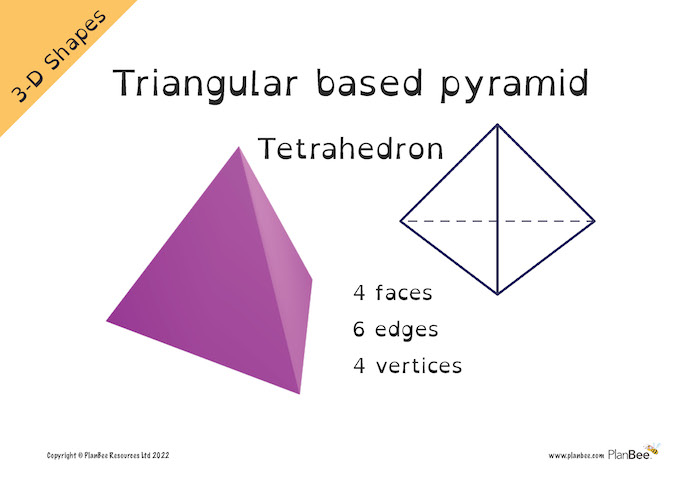## Properties of a square based pyramid

• A square based pyramid is a three-dimensional shape.
• A square based pyramid has 5 faces. Four of the faces are flat triangular faces, one of the faces is a square.
• A square based pyramid has 5 vertices.
• A square based pyramid has 8 edges.
• A square based pyramid is a polyhedron.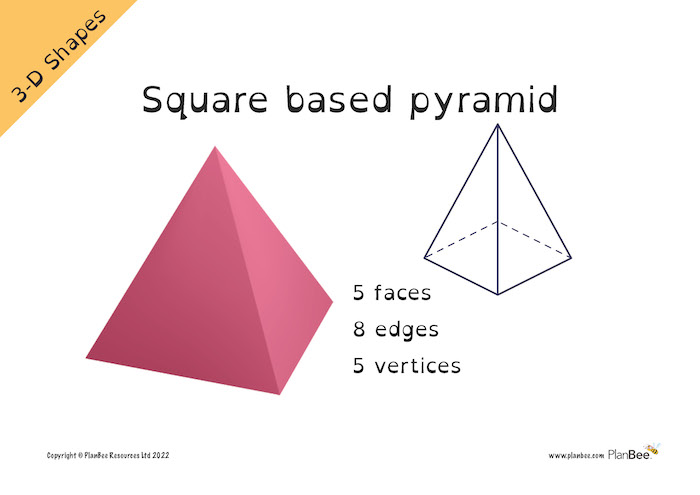## Properties of a rectangular based pyramid

• A rectangular based pyramid is a three-dimensional shape.
• A rectangular based pyramid has 5 faces. Four of the faces are flat triangular faces, one of the faces is a rectangle.
• A rectangular based pyramid has 5 vertices.
• A rectangular based pyramid has 8 edges.
• A rectangular based pyramid is a polyhedron.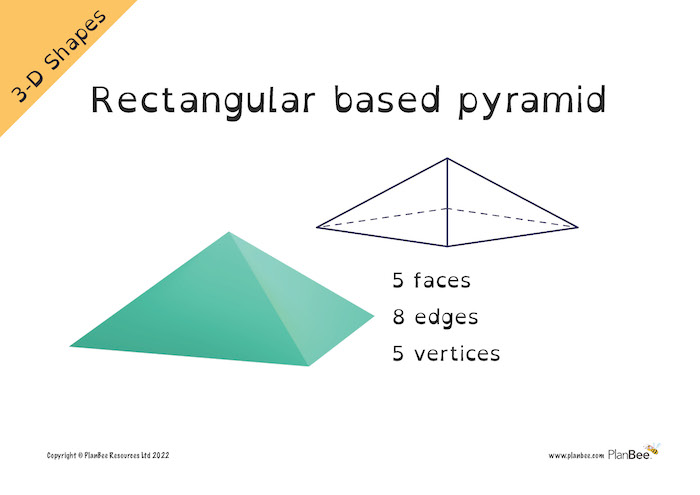## When are 3D shapes taught in the Maths National Curriculum?

3D shapes are taught as part of the geometry strands of the maths curriculum. This strand is geometry - properties of shapes.

3D shapes are first taught in year 1 when children:

- recognise and name common 2-D and 3-D shapes, including: 3-D shapes [for example, cuboids (including cubes), pyramids and spheres].

In Year 2 children:

- identify and describe the properties of 3-D shapes, including the number of edges, vertices and faces

- identify 2-D shapes on the surface of 3-D shapes [for example, a circle on a cylinder and a triangle on a pyramid]

- compare and sort common 2-D and 3-D shapes and everyday objects.

In Year 3 children:

- draw 2-D shapes and make 3-D shapes using modelling materials; recognise 3-D shapes in different orientations and describe them

In Year 5 children:

- identify 3-D shapes, including cubes and other cuboids, from 2-D representations

In Year 6 children:

- recognise, describe and build simple 3-D shapes, including making nets